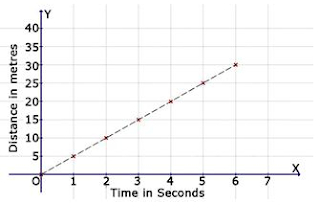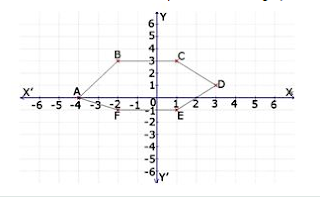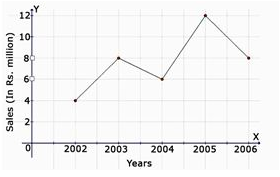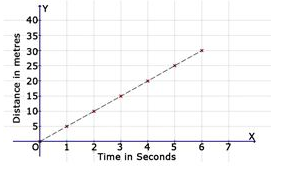>

## MCQ Questions for Class 8 Maths: Ch 15 Introduction to graphs1. Find the distance covered in 6 seconds.(a) 30 m
(b) 35 m
(c) 25 m
(d) none of these
► (a) 30 m

2. Find the distance covered in 5 seconds.(a) 25 m
(b) 10 m
(c) 20 m
(d) none of these
► (a) 25 m

3. Find the coordinates of the point E from the graph.(a) (1, -1)
(b) (-4, 5)
(c) (0, -4)
(d) None of these
► (a) (1, -1)

4. Find the coordinates of the point F from the graph.(a) (-4, 5)
(b) (-2, -1)
(c) (0, -4)
(d) None of these
► (b) (-2, -1)

5. Find the distance covered in 2 seconds.(a) 15 m
(b) 20 m
(c) 25 m
(d) 10 m
► (d) 10 m

6. On which axis does the point (0, 5) lie?
(a) origin
(b) x-axis
(c) y-axis
(d) None of these
► (c) y-axis

7. Which of the points given is a point on the X-axis?
(a) (5,0)
(b) (0,5)
(c) (5,3)
(d) (3,5)
► (a) (5,0)

8. Find the coordinates of the point C from the graph.(a) (1, 3)
(b) (-4, 5)
(c) (0, -4)
(d) None of these
► (a) (1, 3)

9. In which quadrant does the point Q (-2, -6) lie?
(a) I
(b) II
(c) IV
(d) III
► (d) III

10. On which axis does the point (-1, 0) lie?
(a) x-axis
(b) origin
(c) y-axis
(d) None of these
► (a) x-axis

11. The line graph shows the yearly sales figure for a manufacturing company. From the graph, what were the sales in 2006?
(a) Rs 8 millions
(b) Rs 4 millions
(c) Rs 3 millions
(d) Rs 5 millions
► (a) Rs 8 millions

12. The line graph shows the yearly sales figure for a manufacturing company. From the graph, what were the sales in 2004?(a) Rs 5 millions
(b) Rs 3 millions
(c) Rs 4 millions
(d) Rs 6 millions
► (d) Rs 6 millions

13. Which of the following statements is true?
(a) The X-axis is a vertical line.
(b) The Y-axis is a horizontal line.
(c) The scale on both the axes must be the same in a Cartesian plane.
(d) The point of intersection between the X-axis and Y-axis is called the origin.
► (d) The point of intersection between the X-axis and Y-axis is called the origin.

14. Find the time taken by a body to cover 5 metres.(a) 3 seconds
(b) 4 seconds
(c) 5 seconds
(d) 1 second
► (d) 1 second

15. Find the coordinates of the point A from the graph.
(a) (-4, 5)
(b) (-4, 0)
(c) (0, -4)
(d) none of these
► (b) (-4, 0)

16. Find the distance covered in 7 seconds.(a) 30 m
(b) 25 m
(c) 35 m
(d) 20 m
► (c) 35 m